﻿ Quadratic Yield Response of Methane Gas to Input Ratio of Organic Loading Rate and Hydraulic Retention Time during Microbial Digestion of Fruit Wastes

C. I. Nwoye, I. C. E. Umeghalu, S. E. Ede, N. I. Amalu, W. C. Onyia, N. E. IdenyiOPEN ACCESSPEER-REVIEWED

Quadratic Yield Response of Methane Gas to Input Ratio of Organic Loading Rate and Hydraulic Retention Time during Microbial Digestion of Fruit Wastes

C. I. Nwoye1,, I. C. E. Umeghalu2, S. E. Ede3, N. I. Amalu4, W. C. Onyia3, N. E. Idenyi5

1Department of Metallurgical and Materials Engineering, Nnamdi Azikiwe University, Awka, Nigeria

2Department of Agric and Bioresoures Engineering

3Department of Metallurgical and Materials Engineering, Enugu State University of Science & Technology, Enugu, Nigeria

4Project Development Institute Enugu

5Department of Industrial Physics, Ebonyi State University, Abakiliki, Nigeria

Abstract

The yield response of methane gas during microbial digestion of fruit wastes was evaluated based on the operational input ratio of organic loading rate (OLR) and hydraulic retention time (HRT). Computational analysis of generated experimental results indicates that the yield response was empirically a two-factorial quadratic model which was validated for predictive analysis and evaluation. The validity of the model; ζ = 2.1863 (ϑ/ɤ)2 - 2.4573 (ϑ/ɤ) + 0.72 was rooted on the core model expression ζ - 0.72 = 2.1863(ϑ/ɤ)2 – 2.4573 (ϑ/ɤ) where both sides of the expression are correspondingly approximately equal. Results of methane gas yield were generated using regression model and its trend of distribution was compared with that from derived model for the purpose of verifying its validity relative to experimental results. The results of the verification process show very close dimensions of covered areas and shapes designating methane gas yield, which precisely translated into significantly similar trend of data point’s distribution for experimental (ExD), derived model (MoD) and regression model-predicted (ReG) results. Methane gas yield per unit input ratio OLR/ HRT were evaluated from experimental, derived model & regression model predicted results as 1.0035, 0.9893 & 0.9574 m6 Kg -2 d2 respectively. Standard errors incurred in predicting methane gas yield for each value of OLR, HRT & OLR/HRT considered as obtained from experiment, derived model and regression model were 0.1237, 0.1032 & 0.0226%, 0.1214, 0.1055 & 0.0221 % and 0.122, 0.1032 & 2.5336 x 10-5 respectively. The operationally viable deviation range of model-predicted methane gas yield from the experimental results was 3.75-15.25 %. This translated into 84.75-96.26 % operational confidence and reliability level for the derived models, as well as 0.85–0.96 yield response coefficient of methane gas to the input ratio OLR/ HRT.

At a glance: Figures

1234
Prev Next

• Nwoye, C. I., et al. "Quadratic Yield Response of Methane Gas to Input Ratio of Organic Loading Rate and Hydraulic Retention Time during Microbial Digestion of Fruit Wastes." Biomedicine and Biotechnology 2.4 (2014): 93-101.
• Nwoye, C. I. , Umeghalu, I. C. E. , Ede, S. E. , Amalu, N. I. , Onyia, W. C. , & Idenyi, N. E. (2014). Quadratic Yield Response of Methane Gas to Input Ratio of Organic Loading Rate and Hydraulic Retention Time during Microbial Digestion of Fruit Wastes. Biomedicine and Biotechnology, 2(4), 93-101.
• Nwoye, C. I., I. C. E. Umeghalu, S. E. Ede, N. I. Amalu, W. C. Onyia, and N. E. Idenyi. "Quadratic Yield Response of Methane Gas to Input Ratio of Organic Loading Rate and Hydraulic Retention Time during Microbial Digestion of Fruit Wastes." Biomedicine and Biotechnology 2, no. 4 (2014): 93-101.

 Import into BibTeX Import into EndNote Import into RefMan Import into RefWorks

1. Introduction

The renewed awareness and growing demand for a sustainable application of renewable energy for domestic use and industrial growth has resulted to successful processes of conversion of animal dung, fruits and vegetable wastes to biogases used for heat and power generation.

Research  has reported an optimum biogas production at carbon: nitrogen ratio, 25–30 : 1. Report  has shown that the optimum loading rate of the feedstock varied as per its nature, and likewise HRT varied as per the loading rate. Past report  indicates that an HRT of 14 days was optimum for biogas production from cow dung. Similar report  shows that 15 d is the optimum retention time for the production of biogas from cattle dung. The report stated that this retention time was the best for maximum production of biogas from cow dung. Further observations revealed that shorter HRT resulted in accumulation of VFA, whereas at HRT longer than 15 d the digester components were not fully utilized. Research  carried out to investigate the influence of varying TS content on biogas production revealed that optimum production was observed at 8 per cent TS. However, it was observed that methane content of the produced biogas was did not vary significantly with varying levels of TS. Hence, they suggested that high TS content of 14% cattle dung (2: 1 dilution) can be used in areas during the time of water scarcity rather than discontinuing the feeding of the digester.

Scientists  have evaluated the applicability of various substrates for biogas production. The results of the evaluation indicate that the two most important parameters in the selection of particular plant feed stocks are the economic considerations and the yield of methane for fermentation of that specific feedstock. The researchers reported that the highest yield of methane was from root crops followed by forage grasses, and fresh-water aquatics. The deduction followed assessment of methane yield from fresh-water aquatics, forage grasses, roots and tubers, and marine species. Further research  posited that marine species give the lowest yield of methane. Studies  on the kinetics of methane yield show that the yield is generally higher in leaves than in stems. This concept is line with the results derived from application of Ipomoea fistulosa as substrate for methane production by other researcher [9, 10].

Studies  on biogas production from food industry wastes and varieties of biomass materials  have shown that pretreatment of feedstock improved the biogas yield and its methane gas content. It was observed  that though the agricultural residues, such as straw, need to be reduced to a particle size of 0.4 mm for better biogas production, succulent leaves such as Mirabilis sp., Ipomoea fistulosa, etc. can be fed without any shredding. Evaluations of biogas production from Gliricidia sp., Albizia sp., and Parthenium sp. in plug flow digester  indicates improved gas yield with specific inoculum. It was reported  that pretreatment with NaOH increased the gas production from parthenium-fed digesters (0.46 mm– 3 d– 1 and the methane yield was 0.11 mkg– 1 VS). Earlier studies [15, 16] evaluated the applicability of water hyacinth as alternate substrate together with cattle waste for biogas digesters. Scientist  have developed horizontal plug flow (PF) digester and solid state stratified bed (SSB) digester for bio-methanation of biomass and observed that SSB performed better in terms of stability and gas production.

Following evaluation of various kinds of fruit wastes as potential ingredients for methane production , it was found that tomato, mango, pineapple, lemon, and orange processing waste, yielded 0.62, 0.56, 0.77, 0.72 and 0.63 m3 of biogas/kg of VS respectively. It was established  that Mango peel supplemented with urea to adjust the C: N ratio to 20–30 : 1 resulted in the stability of the digester. Addition of nitrogen in the form of silkworm waste and oilseed extracts, such as neem and castor, has been shown  to increase the methane content of the biogas produced. Performance analysis of biogas digester indicates that the digester is stable at a loading rate of 3.8 kg VS m–3 d– 1 . This followed an investigation on the influence of successive addition of fruit and vegetable solid wastes during biogas production. Further observations indicate that no noticeable changes in the rates and yields of biogas occurred as a result of minor manipulation in nutritional and operational parameters which practically affected the general functioning and output of the digester fed with different fruits and vegetable wastes (mango, pineapple, tomato, jack fruit, banana, and orange) for a considerably long time.

Biogas production  using mango peel (on a Pilot plant of volumetric capacity 1.5 m3 and digester type KVIC) significantly showed that supplementation with essential nutrients improved the digestibility of feedstock, yielding as high as 0.6 m3/kg VS with a biogas content of 52% at a loading rate of 8–10%. Furthermore, addition of sugarcane filter mud at a rate of 200 g/4 kg of mango peel in 1.5 m3 digester increased biogas yield substantially with a methane content of 60%. Reported  also indicated that addition of extract of nirmali seeds, hybrid beans, black gram, and guar gum seeds (as additives) at 2–3% level increased the biogas production significantly. This increment was attributed to the galactomannan constituent of the leguminous seeds which increased the floc formation, thereby retaining the organisms in the digester.

An evaluation of methane gas yield was carried out  based on the hydraulic retention time (HRT) of waste fruits undergoing biodegradation. The empirical assessment was carried out using a derived and validated model expressed as;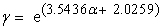(1)

The model depicts an exponential relationship between methane yield and the HRT. Statistical analysis of the model-predicted and experimental gas methane yield for each value of HRT considered shows a standard error of 0.0081 and 0.0114% respectively. Furthermore, the precise correlation between methane yield and HRT as obtained from derived model and experimental results were evaluated as 0.9716 and 0.9709 respectively. Methane gas yield per unit HRT as obtained from derived model and experiment are 0.0196 and 0.0235 (m3 kg−1 VS) days−1 respectively.

The maximum deviation of the model-predicted methane yield from associated corresponding experimental value was found to be less than 16%. It was also found that the validity of the model is rooted on the expression 0.2822 lnγ = α + 0.5717 where both sides of the expression are correspondingly approximately equal.

Predictive analysis of methane gas yield during bio-treatment of fruit wastes in the digester has been carried out  using a derived model. It was found that the validity of the model expressed as: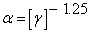(2)

was rooted on the core expression (Log α)/N = Log (γ)-1 where both sides of the expression are correspondingly approximately equal. The overall validity of the derived model was established using the 4th Degree Model Validity Test Technique (4th DMVTT) which are computational, graphical, statistical and deviational analysis. Computational analysis of the model-predicted and experimental results indicates that methane gas yield per unit organic loading rate are - 0.0421 and- 0.0395 (VS)2 d-1 as obtained from experiment and derived model respectively. The graphical analysis, apart from showing very close alignment of curves from both experimental and model-predicted results, indicates 0.9923 and 1.0000 as the correlation between methane yield and organic loading rate as obtained from experiment and derived model are respectively. Statistical analysis of the results indicate that the variance and standard deviation as obtained from experiment and derived model are 9.1004 x 10-4 and 0.0302 as well as 8.0934 x10-4 and 0.0284 respectively, indicating proximate agreement. Deviational analysis indicates that the maximum deviation of the model-predicted methane yield from the corresponding experimental value is less than 6%.

The reliability level of methane gas production dependence on organic loading rate and hydraulic retention time during biodegradation of fruit wastes has been assessed . A two-factorial linear model was derived and validated for the predictive analysis and evaluation. The validity of the model;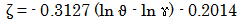(3)

was rooted on the core model expression ζ + 0.2014 = - 0.3127(ln ϑ - ln ɤ) where both sides of the expression are correspondingly approximately equal. Regression model was used to generate results of methane gas yield, and its trend of distribution was compared with that from derived model as a means of verifying its validity relative to experimental results. The results of this verification show very close dimensions of aligned areas designating methane gas yield, which precisely translated into significantly similar trend of data point’s distribution for experimental (ExD), derived model (MoD) and regression model-predicted (ReG) results. Evaluations from generated results indicated that methane gas yield per unit hydraulic retention time and organic load mass as obtained from experiment, derived model & regression model were 0.0242, 0.0239 & 0.0209 m3 kg -1 VS d-1 and 0.0064, 0.0063 & 0.0055 (m3 kg-1)2 respectively. Standard errors incurred in predicting the methane gas yield for each value of the hydraulic retention time and organic loading rate considered as obtained from experiment, derived model & regression model were 0.1032, 0.1042, & 0.0011 % and 0.1237, 0.1220 & 2.5x10-5 % respectively. Deviational analysis indicates that the maximum deviation of model-predicted methane gas yield from the experimental results is less than 22%. This translates into over 78% operational confidence and reliability level for the derived model as well as over 0.78 reliability coefficient for the methane gas production dependence on organic loading rate and hydraulic retention time.

The present work aims at establishing an empirical methane gas yield response expression based on the input ratio of organic loading rate and hydraulic retention time during microbial digestion of fruit wastes. The derived empirical expression will be validated and used for the future predictive assessment.

2. Materials and Methods

A weighed quantity of prepared fruit wastes was put in the digested containing the appropriate microbes. Details of the experimental procedure and associated process conditions are as stated in the past report .

Table 1. Variation of methane gas yield with organic loading rate (OLR), hydraulic retention time (HRT) and input ratio OLR/ HRT 

Where

(ɤ) = Hydraulic retention time (HRT) (days)

(ζ ) = Methane gas production (m3 kg-1 VS)

(ϑ/ɤ)= OLR/ HRT ratio

2.1. Model Formulation

Experimental data obtained from research work  were used for this work. Computational analysis of the data  shown in Table 1, gave rise to Table 2 which indicate that;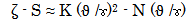(4)

Introducing the values of S, K and N into equation (4) reduces it to;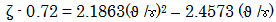(5)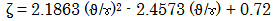(6)

S = 0.72, K = 2.1863 and N = 2.4573 are empirical constants (determined using C-NIKBRAN .

3. Boundary and Initial Condition

Consider prepared fruit wastes (in a digester) interacting with microbes. The digester atmosphere was not contaminated i.e (free of unwanted gases and dusts). Range of organic loading rate, hydraulic retention time and input ratio OLR/ HRT used: 3.8-7.6 Kg VS m-3 d-1, 8 – 20 days and 0.19 – 0.475 respectively. Mass of wastes used, resident time, treatment temperature, growth rate of microbes and other process conditions are as stated in the experimental technique .

The prevailed boundary conditions are: anaerobic atmosphere to enhance bacterial action on the wastes (since the digester was air-tight closed). At the bottom of the particles, a zero gradient for the gas scalar are assumed and also for the gas phase at the top of the waste particles. The biodegraded waste was stationary. The sides of the waste particles are taken to be symmetries.

4. Results and Discussion

4.1. Model Validation

Equation (6) is the derived model.

Table 2. Variation of ζ - 0.72 with 2.1863(ϑ /ɤ)2 – 2.4573 (ϑ /ɤ)

The validity of the model is strongly rooted on equation (4) where both sides of the equation are correspondingly approximately equal. Table 2 also agrees with equation (4) following the values of ζ - 0.72 and 2.1863(ϑ/ɤ)2 -2.4573 (ϑ/ɤ) evaluated from the experimental results in Table 1. Furthermore, the derived model was validated by comparing the methane gas yield predicted by the model and that obtained from the experiment . This was done using the 4th Degree Model Validity Test Techniques (4th DMVTT); computational, graphical, statistical and deviational analysis .

4.2. Statistical Analysis

Standard Error (STEYX)

The standard errors incurred in predicting methane gas yield for each value of OLR, HRT & OLR/HRT considered as obtained from experiment and derived model were 0.1237, 0.1032 & 0.0226% and 0.1214, 0.1055 & 0.0221 % respectively. The standard error was evaluated using Microsoft Excel version 2003.

Figure 1. Coefficient of determination between methane gas yield and organic loading rate as obtained from experiment 
Figure 3. Coefficient of determination between methane gas yield and hydraulic retention time as obtained from experiment 
Figure 4. Coefficient of determination between methane gas yield and hydraulic retention time as predicted by derived model
Figure 5. Coefficient of determination between methane gas yield and organic loading rate- hydraulic retention time ratio as obtained from experiment 
Figure 6. Coefficient of determination between methane gas yield and organic loading rate- hydraulic retention time ratio as predicted by derived model

Correlation (CORREL)

The correlation coefficient between methane gas yield and OLR, HRT & OLR/HRT were evaluated (using Microsoft Excel Version 2003) from results of the experiment and derived model. These evaluations were based on the coefficients of determination R2 shown in Figure 1-Figure 6.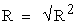(7)

The evaluated correlations are shown in Table 3-Table 5. These evaluated results indicate that the derived model predictions are significantly reliable and hence valid considering its proximate agreement with results from actual experiment.

Table 5. Comparison of the correlations evaluated from derived model predicted and experimental results based on input ratio OLR/ HRT

4.3. Graphical Analysis

Comparative graphical analysis of Figure 7-Figure 9 show very close alignment of the curves and shapes from the experimental (ExD) and model-predicted (MoD) methane gas yields. Furthermore, the degree of alignment of these curves is indicative of the proximate agreement between both experimental and model-predicted methane gas yields.

Figure 7. Comparison of methane gas yields (relative to organic loading rate) as obtained from experiment  and derived model
Figure 8. Comparison of area covered by methane gas yields (relative to hydraulic retention time) as obtained from experiment  and derived model
Figure 9. Comparison of methane gas yields (relative to organic loading rate- hydraulic retention time ratio) as obtained from experiment  and derived model
4.4. Comparison of Derived Model with Standard Model

The validity of the derived model was also verified through application of the regression model (Reg) (Least Square Method using Excel version 2003) in predicting the trend of the experimental results. Comparative analysis of Figure 10-Figure 12 shows very close dimensions of aligned areas covered by methane gas yield, which precisely translated into significantly similar trend of data point’s distribution for experimental (ExD), derived model (MoD) and regression model-predicted (ReG) results of methane gas yield.

Figure 10. Comparison of methane gas yield (relative to organic loading rate) as obtained from experiment , derived model and regression model
Figure 11. Comparison of areas covered by methane gas yield (relative to hydraulic retention time) as obtained from experiment , derived model and regression model
Figure 12. Comparison of methane gas yield (relative to organic loading rate- hydraulic retention time ratio) as obtained from experiment , derived model and regression model

Also, the calculated correlations (from Figure 10-Figure 12) between methane gas yield and OLR, HRT & OLR/HRT for results obtained from regression model were 0.9734, 0.9734 & 1.0000 respectively. These values are in proximate agreement with both experimental and derived model-predicted results. The standard errors incurred in predicting methane gas yield for each value of OLR, HRT & OLR/HRT considered as obtained from regression model were 0.1220, 0.1032 & 2.5336 x 10-5 % respectively.

Computational Analysis

Critical computational analysis of the experimental and model-predicted methane gas yield was carried out to ascertain the degree of validity of the derived model. This was done by comparing results of evaluated methane gas yield per unit value of input ratio OLR/ HRT as obtained from experimental and derived model within organic loading rate and hydraulic retention time range: 3.8-7.6 kg VS m-3 d-1 and 8-20 days respectively.

Methane gas yield per unit OLR /HRT ratio ζ / (ϑ /ɤ) (m6 Kg -2 d2) was calculated from the equation;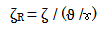(8)

Re-written as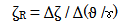(9)

Equation (9) is detailed as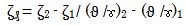(10)

Where

Δζ = Change in the methane gas yield ζ 2, ζ 1 at two values of OLR/ HRT ratios (ϑ /ɤ)2, (ϑ /ɤ)1.

Considering the points (0.19, 0.32) & (0.475, 0.03), (0.19, 0.332) & (0.475, 0.0461) and (0.19,0.3022) & (0.475, 0.0255) as shown in Table 1 and Figure 12, then designating them as (ζ1, (ϑ /ɤ) 1) & (ζ2, (ϑ /ɤ) 2) for experimental, derived model and regression model predicted results respectively, and then substituting them into equation (10), gives the slopes: 1.0035, 0.9893 and 0.9574 (m6 Kg -2 d2) respectively as their corresponding methane gas yield per unit input ratio OLR/ HRT.

The proximity between these values indicates significantly high validity level for the derived model.

Deviational Analysis

Analysis of the methane gas yields precisely obtained from experiment  and derived model shows deviations on the part of the model-predicted values relative to values obtained from the experiment. This is attributed to the fact that the surface properties of fruit wastes and the physiochemical interactions between the fruit wastes and the infesting microbes which played vital roles during the biogas production process  were not considered during the model formulation. This necessitated the introduction of correction factor, to bring the model-predicted methane gas yield to those of the corresponding experimental values.

The deviation Dv, of model-predicted methane gas yield from the corresponding experimental result was given by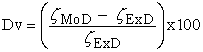(11)

Where

ζExD and ζMoD are methane gas yield evaluated from experiment and derived model respectively.

The correction factor took care of the negligence of operational contributions of surface properties of the fruit wastes and the physiochemical interactions between the fruit wastes and infesting microbes which actually played vital role during the methane gas production process. The model predicted results deviated from those of the experiment because these contributions were not considered during the model formulation. Introduction of the corresponding values of Cf from equation (12) into the model gives exactly the corresponding experimental methane gas yield.

Table 6. Variation of deviation and correction factor with input ratio OLR /HRT

Deviational analysis of Table 6 relative to input ratio OLR/HRT indicates that the operationally viable deviation range of model-predicted methane gas yield from the experimental results is 3.75-15.25 %. This translated into 84.75-96.26 % operational confidence and reliability level for the derived models as well as 0.85–0.96 reliability coefficient for the yield response of methane gas to input ratio OLR/ HRT.

Consideration of equation (11) and critical analysis of Table 6 and Figure 12 indicate that the highlighted deviation range corresponds to methane gas yields: 0.0461- 0.332 m3 kg -1 VS and input ratio OLR/HRT: 0.19-0.475 m6 Kg -2 d2 respectively.

Correction factor, Cf to the model-predicted results was given by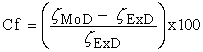(12)

Critical analysis of Table 6 indicates that the evaluated correction factors are negative of the deviation as shown in equations (11) and (12). Table 6 shows that the operationally viable range of correction factors to the model-predicted methane gas yield were - 3.75 to -15.25 %. Table 6 and Figure 12 indicate that these highlighted correction factors correspond to methane gas yields: 0.0461- 0.332 m3 kg -1 VS and input ratio OLR/HRT: 0.19-0.475 m6 Kg -2 d2 respectively.

It is important to state that the deviation of model predicted results from that of the experiment is just the magnitude of the value. The associated sign preceding the value signifies that the deviation is a deficit (negative sign) or surplus (positive sign).

5. Conclusion

The yield response of methane gas during microbial digestion of fruit wastes was evaluated based on the operational input ratio of organic loading rate (OLR) and hydraulic retention time (HRT). Computational analysis of experimental results of methane gas yield generated during microbial digestion of fruit wastes indicates that the yield response is empirically a two-factorial quadratic model which was validated for predictive analysis and evaluation. The validity of the model was rooted on the core model expression ζ - 0.72 = 2.1863(ϑ/ɤ)2 – 2.4573 (ϑ/ɤ) where both sides of the expression are correspondingly approximately equal. Methane gas yield per unit input ratio OLR/ HRT were evaluated from experimental, derived model & regression model predicted results as 1.0035, 0.9893 & 0.9574 m6 Kg-2 d2 respectively. Standard errors incurred in predicting methane gas yield for each value of OLR, HRT & OLR/HRT considered as obtained from experiment, derived model and regression model were 0.1237, 0.1032 & 0.0226%, 0.1214, 0.1055 & 0.0221 % and 0.122, 0.1032 & 2.5336 x 10-5 respectively. The operationally viable deviation range of model-predicted methane gas yield from the experimental results was 3.75-15.25 %. This translated into 84.75-96.26 % operational confidence and reliability level for the derived models, as well as 0.85–0.96 yield response coefficient of methane gas to the input ratio OLR/ HRT.

References

  Mital, K. M. (1996). Biogas Systems: Principles and Applications, New Age International (P) Limited Publishers, New Delhi, p. 412.In article  Ramasamy, K. (1998). in Renewable Energy – Basics and Technology (ed. Gupta, C. L.), Auroville Foundation and Solar Agni International, Pondicherry, pp. 239-271.In article  Yeole, T. Y. and Ranade, D. R. (1992). Alternative Feedstocks for Biogas, 10-16.In article  Gadre, R. V., Ranade, D. R. and Godbole, S. H. (1990) Indian J. Environ. Hlth., 32, 45-49.In article  Ranade, D. R., Nagarwala, N. N., Dudhbhate, J. A., Gadre, R. V. and Godbole, S. H. (1990). Indian J. Environ. Hlth., 32, 63-65.In article  Smith, W. H., Wilkie, A. C. and Smith, P. H.(1992). TIDE, 2, 1-20.In article  Smith, P. H., Bordeaux, F. M., Wilkie, A., Yang, J., Boone, D., Mah, R. A., Chynoweth, D. and Jerger, D. (1998). in Methane from Biomass: A Systems Approach (eds Smith, W. H. and Frank, J. R.), Elsevier Applied Science, London, p. 500.In article PubMed  Chynoweth, D. P., Turik, C. E., Owens, J. M., Jerger, D. E. and Peck, M. W.(1993). Biomass Bioenergy, 5, 95-111.In article CrossRef  Sharma, S. K., Mishra, I. M., Sharma, M. P. and Saini, J. S. (1998). Biomass, 17, 251-263.In article CrossRef  Sharma, S. K., Saini, J. S., Mishra, I. M. and Sharma, M. P.(1989). Biol. Wastes, 28, 25-32.In article CrossRef  Krishnanand, K. (1994). Indian Food Ind., 13, 33-35.In article  Gunaseelan, V. N. (1997). Biomass Bioenergy, 13, 83-114.In article CrossRef  Ramasamy, K., Ilamurugu, K., Sahul Hameed, M. and Maheswari, M. (1995). Tech. Bull., 5, 36.In article  Gunaseelan, V. N. (1994). Biomass Bioenergy, 6, 391-398.In article CrossRef  Deshpande, P., Sarnaik, S., Godbole, S. H. and Wagle, P. M. (1979). Curr. Sci., 48, 490-492.In article  Mallick, M. K., Singh, U. K. and Ahmad, N. (1990). Biol. Wastes, 31, 315-319.In article CrossRef  Chanakya, H. N., Ganguli, N. K., Anand, V. and Jagadeesh, K. S. (1995). Energy Sustain. Dev., 1, 43-46.In article  Anonymous, (1989). Final report submitted to Department of Non-Conventional Energy Sources, Government of India, New Delhi.In article  Viswanath, P., Devi, S. and Krishnanand., Biores. Technol., 1992, 40, 43-48.In article CrossRef  Nwoye, C. I., Asuke, F., Ijomah, A., Obiorah, S.(2012). Model for Assessment Evaluation of Methane Gas Yield Based on Hydraulic Retention Time during Fruit Wastes Biodigestion. Journal of Minerals and Materials Characterization and Engineering, 11: 947-952.In article  Nwoye, C. I., and Nwabanne, J. T. (2013). Empirical analysis of methane gas yield dependence on organic loading rate during microbial treatment of fruit wastes in digester. Advances in Applied Science Research, 2013, 4(1):308-318.In article  C. I. Nwoye, A. O. Agbo, K. C. Nnakwo, E. M. Ameh, and C. C. Nwogbu, “Reliability Level of Methane Gas Production Dependence on Organic Loading Rate and Hydraulic Retention Time during Biodegradation of Fruit Wastes.” International Journal of Environmental Bioremediation & Biodegradation 1, no. 2 (2013): 66-72.In article  Nwoye, C. I., (2008). Data Analytical Memory; C-NIKBRAN.In article PubMed  Nwoye, C. I., and Nwabanne J. T. (2013). Empirical Analysis of Methane Gas Yield Dependence on Organic Loading Rate during Microbial Treatment of Fruit Wastes in Digester. Advances in Applied Science Research 4(1): 308-318.In article